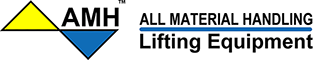Lever Chain Hoists LA Series | 48WS

# Ecommerce Websites, Catalogs, and Marketing Services

## We Do Not Sell Products

BROWSE CATEGORIES

Search:

# Lever Chain Hoists LA Series

## All Materials Handling, Inc.SKUInfo/Size
LA008
US Ton = 43163, Chain = C6X18Z, Parts = 1 , A inch = 13.4, B inch = 3.9, C inch = 5.2, D inch = 10.5, E inch = 1.02, F inch = 0.98, G inch = 6.2, P @WLL = 47, W /ft.lift0.54, W 10ft.lift = 14.9
LA010
US Ton = 1, Chain = C6X18Z, Parts = 1 , A inch = 13.4, B inch = 3.9, C inch = 5.2, D inch = 12.1, E inch = 1.02, F inch = 0.98, G inch = 6.2, P @WLL = 51, W /ft.lift0.54, W 10ft.lift = 15.3
LA016
US Ton = 1.75, Chain = C7X21Z, Parts = 1 , A inch = 15.7, B inch = 4.4, C inch = 5.7, D inch = 14, E inch = 1.14, F inch = 1.18, G inch = 7.2, P @WLL = 66, W /ft.lift0.74, W 10ft.lift = 24.1
LA020
US Ton = 2.25, Chain = C8X24Z, Parts = 1 , A inch = 18.1, B inch = 4.6, C inch = 7.6, D inch = 14, E inch = 1.38, F inch = 1.3, G inch = 8.1, P @WLL = 66, W /ft.lift0.92, W 10ft.lift = 36.2
LA025
US Ton = 2.75, Chain = C9X27Z, Parts = 1 , A inch = 19.1, B inch = 4.6, C inch = 7.6, D inch = 14, E inch = 1.38, F inch = 1.3, G inch = 8.1, P @WLL = 74, W /ft.lift1.25, W 10ft.lift = 37.9
LA032
US Ton = 3.5, Chain = C10X28Z, Parts = 1 , A inch = 20.5, B inch = 4.8, C inch = 7.8, D inch = 16.5, E inch = 1.46, F inch = 1.42, G inch = 8.3, P @WLL = 72, W /ft.lift1.5, W 10ft.lift = 44.8
LA063
US Ton = 7, Chain = C10X28Z, Parts = 2 , A inch = 25.2, B inch = 4.8, C inch = 9.1, D inch = 16.5, E inch = 1.69, F inch = 1.69, G inch = 8.3, P @WLL = 74, W /ft.lift3, W 10ft.lift = 66.3
LA090
US Ton = 10, Chain = C10X28Z, Parts = 3 , A inch = 28.7, B inch = 4.8, C inch = 13.3, D inch = 16.5, E inch = 1.73, F inch = 1.73, G inch = 8.3, P @WLL = 76, W /ft.lift4.5, W 10ft.lift = 110.6
Quick Overview
Call Mon. through Fri. 8:00 AM - 4:00 PM PST
Phone: 714-573-4083
Email: sales@48ws.com

Can't find what you want? Give us a call and we can help you find it.
Details
Dual rated with more choices of capacity. Hook blocks are bolted allowing easy dis-assembly for complete hook inspection . Lightweight and durable all steel construction with powder coated finish and plated external components to resist corrosion. Premium Grade 80 Zinc plated alloy load chain manufactured in the USA. Self-adjusting double pawl disk type mechanical load brake insures control. Horseshoe chain guard allows operation in any orientation. Optional Overload Protection is new design one directional clutch that will only slip in the lift direction . Meets: ASME/ANSI B3O.16, OSHA, NASA-STD-8719.9. ATEX rated  II 3 GD c IIB 54º C X for limited use in Hazardous Environments.